# 15.11: Radioactive Decay as a Measure of Age

$$\newcommand{\vecs}{\overset { \rightharpoonup} {\mathbf{#1}} }$$ $$\newcommand{\vecd}{\overset{-\!-\!\rightharpoonup}{\vphantom{a}\smash {#1}}}$$$$\newcommand{\id}{\mathrm{id}}$$ $$\newcommand{\Span}{\mathrm{span}}$$ $$\newcommand{\kernel}{\mathrm{null}\,}$$ $$\newcommand{\range}{\mathrm{range}\,}$$ $$\newcommand{\RealPart}{\mathrm{Re}}$$ $$\newcommand{\ImaginaryPart}{\mathrm{Im}}$$ $$\newcommand{\Argument}{\mathrm{Arg}}$$ $$\newcommand{\norm}{\| #1 \|}$$ $$\newcommand{\inner}{\langle #1, #2 \rangle}$$ $$\newcommand{\Span}{\mathrm{span}}$$ $$\newcommand{\id}{\mathrm{id}}$$ $$\newcommand{\Span}{\mathrm{span}}$$ $$\newcommand{\kernel}{\mathrm{null}\,}$$ $$\newcommand{\range}{\mathrm{range}\,}$$ $$\newcommand{\RealPart}{\mathrm{Re}}$$ $$\newcommand{\ImaginaryPart}{\mathrm{Im}}$$ $$\newcommand{\Argument}{\mathrm{Arg}}$$ $$\newcommand{\norm}{\| #1 \|}$$ $$\newcommand{\inner}{\langle #1, #2 \rangle}$$ $$\newcommand{\Span}{\mathrm{span}}$$$$\newcommand{\AA}{\unicode[.8,0]{x212B}}$$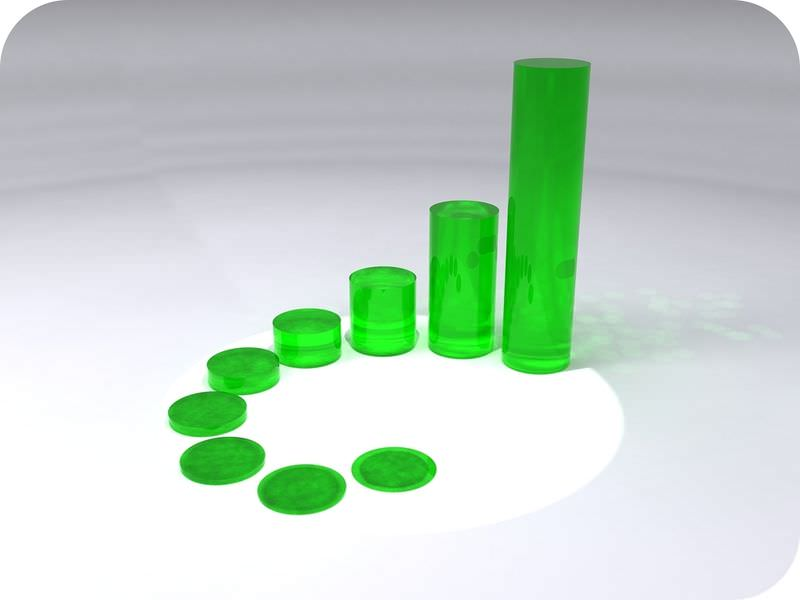When do you stop counting?

Pretend that the large green cylinder is a parent isotope. Now you can visualize the decay of the parent to the daughter. It's easy to see that the second cylinder is half the size of the first. The third is half the size of the second. But when the cylinders get small, the differences are much harder to see. At some point, there is too little of the parent left. That isotope pair is no longer useful for dating.

Radioactive decay is the breakdown of unstable elements into stable elements. To understand this process, recall that the atoms of all elements contain the particles protons, neutrons, and electrons.

Isotopes

An element is defined by the number of protons it contains. All atoms of a given element contain the same number of protons. The number of neutrons in an element may vary. Atoms of an element with different numbers of neutrons are called isotopes.

Consider carbon as an example. Two isotopes of carbon are shown below (Figure below). Compare their protons and neutrons. Both contain six protons. But carbon-12 has six neutrons and carbon-14 has eight neutrons.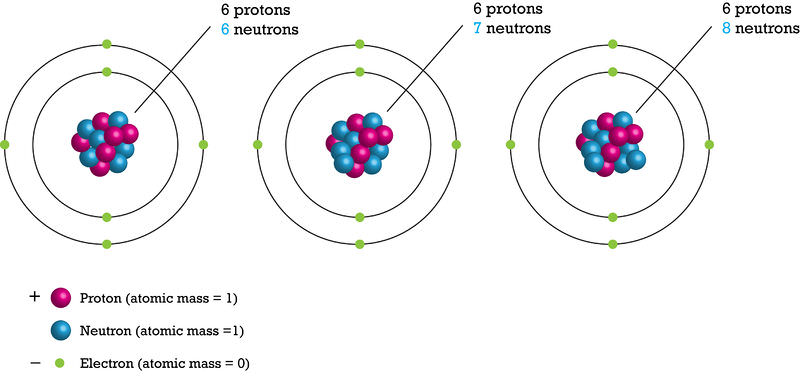Isotopes are named for their number of protons plus neutrons. If a carbon atom had seven neutrons, what would it be named?

Almost all carbon atoms are carbon-12. This is a stable isotope of carbon. Only a tiny percentage of carbon atoms are carbon-14. carbon-14 is unstable. It is a radioactive isotope of carbon. Pictured below is carbon dioxide (Figure below), which forms in the atmosphere from carbon-14 and oxygen. Neutrons in cosmic rays strike nitrogen atoms in the atmosphere. The nitrogen forms carbon-14. Carbon in the atmosphere combines with oxygen to form carbon dioxide. Plants take in carbon dioxide during photosynthesis. In this way, carbon-14 enters food chains.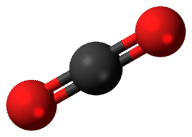Carbon-14 forms in the atmosphere. It combines with oxygen and forms carbon dioxide. How does carbon-14 end up in fossils?

Decay of Unstable Isotopes

Like other unstable isotopes, carbon-14 breaks down, or decays. The original atoms are called the parent isotopes. For carbon-14 decay, each carbon-14 atom loses a beta particle, or a neutron that splits into a proton and an electron. It changes to a stable atom of nitrogen-14. The stable atom at the end is the daughter product (Figure below).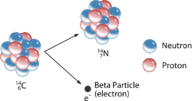Unstable isotopes, such as carbon-14, decay by losing atomic particles. They form different, stable elements when they decay.

The decay of an unstable isotope to a stable element occurs at a constant rate. This rate is different for each parent-daughter isotope pair. The decay rate is measured in a unit called the half-life. The half-life is the time it takes for half of a given amount of an isotope to decay. For example, the half-life of carbon-14 is 5,730 years. Imagine that you start out with 100 grams of carbon-14. In 5,730 years, half of it decays. This leaves 50 grams of carbon-14. Over the next 5,730 years, half of the remaining amount will decay. Now there are 25 grams of carbon-14. How many grams will there be in another 5,730 years? The figure below graphs the rate of decay of a substance (Figure below).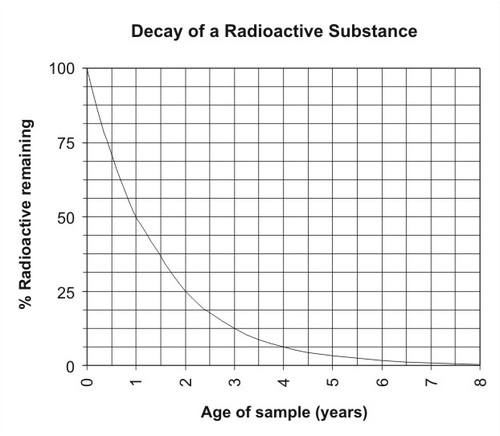The rate of decay of a radioactive substance is constant over time.

## Summary

• A half-life is the time it takes for half of the parent isotopes of an element to change to the daughter product.
• With alpha decay, the nucleus loses two protons and two neutrons.
• Carbon-14 has a half-life of 5,730 years.